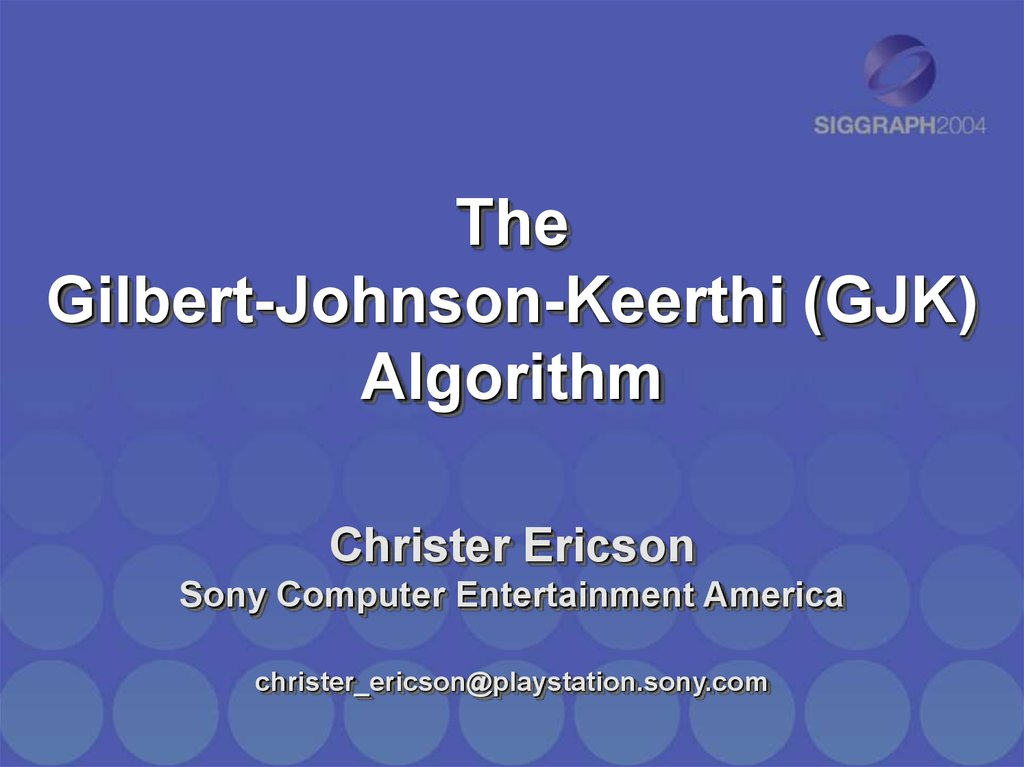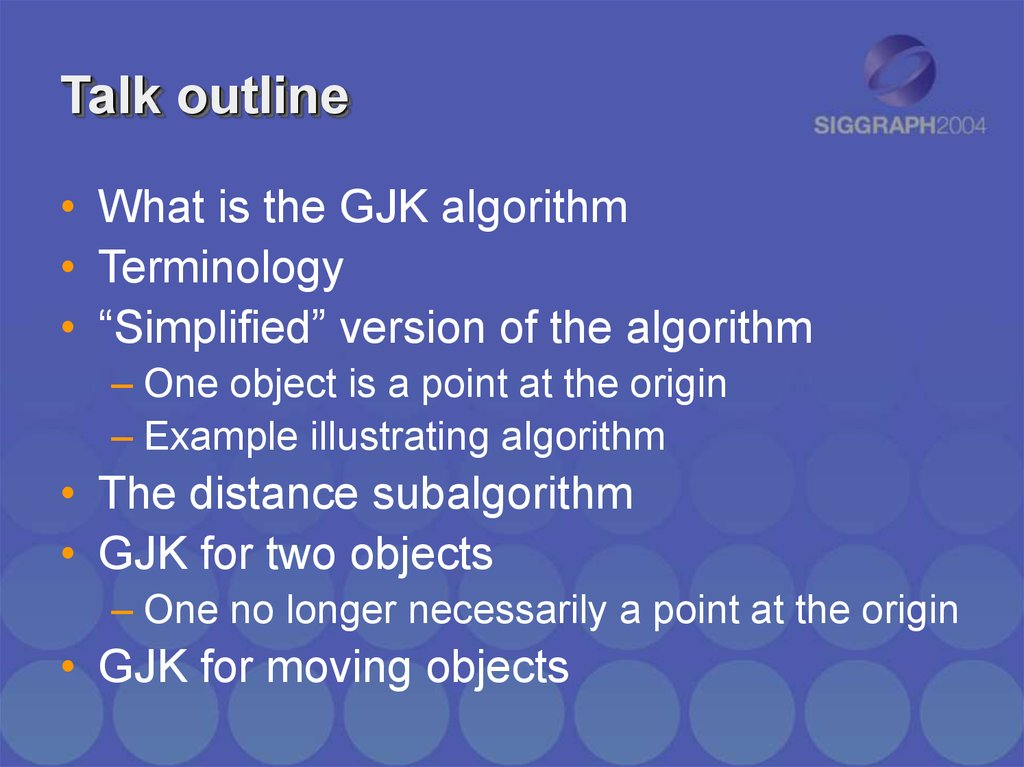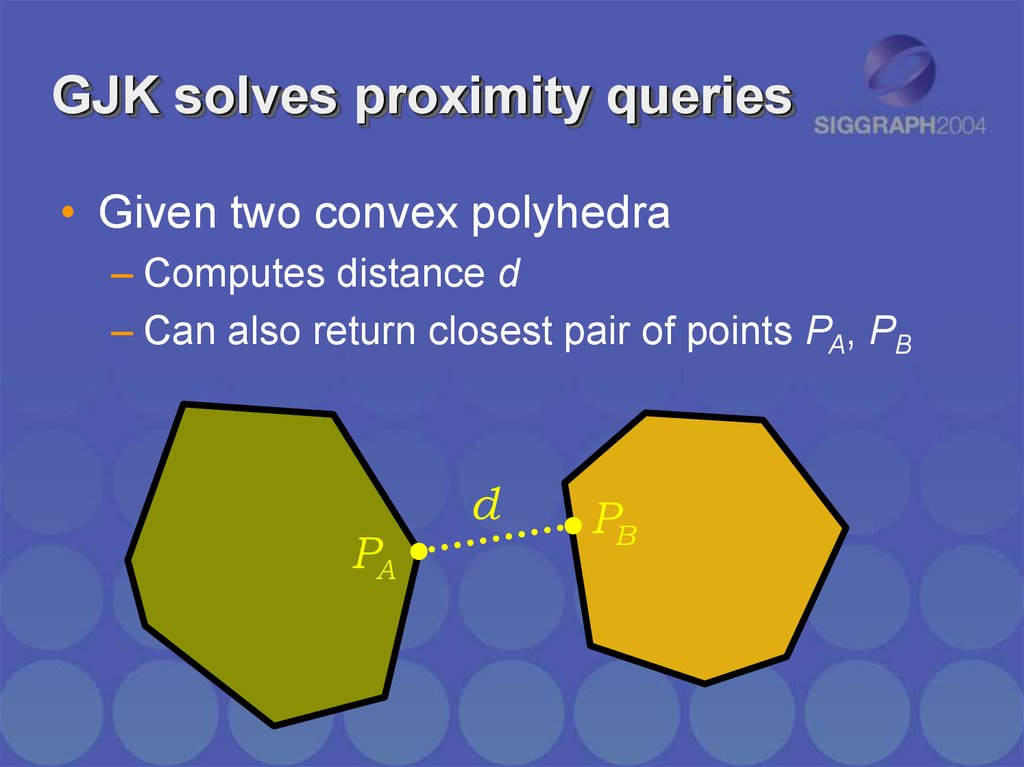# The Gilbert-Johnson-Keerthi (GJK) Algorithm

## 1. The Gilbert-Johnson-Keerthi (GJK) Algorithm

Christer Ericson
Sony Computer Entertainment America
[email protected]

## 2. Talk outline

• What is the GJK algorithm
• Terminology
• “Simplified” version of the algorithm
– One object is a point at the origin
– Example illustrating algorithm
• The distance subalgorithm
• GJK for two objects
– One no longer necessarily a point at the origin
• GJK for moving objects

## 3. GJK solves proximity queries

• Given two convex polyhedra
– Computes distance d
– Can also return closest pair of points PA, PB
PA
d
PB

## 4. GJK solves proximity queries

• Generalized for arbitrary convex objects
– As long as they can be described in terms of
a support mapping function
PA
d
PB

## 5. Terminology 1(3)

P SC (d)
P SC (d)
d
C
C
Supporting (or extreme) point P for direction d
returned by support mapping function SC (d)

0-simplex
1-simplex
2-simplex
simplex
3-simplex

## 7. Terminology 3(3)

Point set C
Convex hull, CH(C)

## 8. The GJK algorithm

1. Initialize the simplex set Q with up to d+1
points from C (in d dimensions)
2. Compute point P of minimum norm in CH(Q)
3. If P is the origin, exit; return 0
4. Reduce Q to the smallest subset Q’ of Q, such
that P in CH(Q’)
5. Let V=SC(–P) be a supporting point in direction
–P
6. If V no more extreme in direction –P than P
itself, exit; return ||P||
7. Add V to Q. Go to step 2

## 9. GJK example 1(10)

INPUT: Convex polyhedron C given as
the convex hull of a set of points
C

## 10. GJK example 2(10)

1.
Initialize the simplex set Q with up to d+1 points from C (in d
dimensions)
Q Q0 ,Q1,Q2
Q1
C
Q2
Q0

## 11. GJK example 3(10)

2.
Compute point P of minimum norm in CH(Q)
Q Q0 ,Q1,Q2
Q1
Q0
P
Q2

## 12. GJK example 4(10)

3.
4.
If P is the origin, exit; return 0
Reduce Q to the smallest subset Q’ of Q, such that P in CH(Q’)
Q Q1,Q 2
Q1
Q0
P
Q2

## 13. GJK example 5(10)

5.
Let V=SC(–P) be a supporting point in direction –P
Q Q1,Q 2
Q1
P
V SC ( P )
Q2

## 14. GJK example 6(10)

6.
7.
If V no more extreme in direction –P than P itself, exit; return ||P||
Add V to Q. Go to step 2
Q Q1,Q2 ,V
Q1
V
Q2

## 15. GJK example 7(10)

2.
Compute point P of minimum norm in CH(Q)
Q Q1,Q2 ,V
Q1
V
P
Q2

## 16. GJK example 8(10)

3.
4.
If P is the origin, exit; return 0
Reduce Q to the smallest subset Q’ of Q, such that P in CH(Q’)
Q Q 2 ,V
V
P
Q2

## 17. GJK example 9(10)

5.
Let V=SC(–P) be a supporting point in direction –P
Q Q 2 ,V
V
P
V ' SC ( P ) Q2

## 18. GJK example 10(10)

6.
If V no more extreme in direction –P than P itself, exit; return ||P||
Q Q 2 ,V
DONE!
V
P
Q2

## 19. Distance subalgorithm 1(2)

• Approach #1: Solve algebraically
– Used in original GJK paper
– Johnson’s distance subalgorithm
Searches all simplex subsets
Solves system of linear equations for each subset
Recursive formulation
From era when math operations were expensive
Robustness problems
– See e.g. Gino van den Bergen’s book

## 20. Distance subalgorithm 2(2)

• Approach #2: Solve geometrically
– Mathematically equivalent
• But more intuitive
• Therefore easier to make robust
– Use straightforward primitives:
• ClosestPointOnEdgeToPoint()
• ClosestPointOnTriangleToPoint()
• ClosestPointOnTetrahedronToPoint()
– Second function outlined here
• The approach generalizes

## 21. Closest point on triangle

• ClosestPointOnTriangleToPoint()
– Finds point on triangle closest to a given point
A
P
Q
B
C

## 22. Closest point on triangle

• Separate cases based on which feature Voronoi
region point lies in
VA
A
E AB
E AC
F
B
VB
E BC
C
VC

AX AB 0
AX AC 0
B
VA
X
A
C

(BC BA ) BA BX 0
AX AB 0
E AB
B
X
A
BX BA 0
C

## 25. GJK for two objects

• What about two polyhedra, A and B?
• Reduce problem into the one solved
– No change to the algorithm!
– Relies on the properties of the
Minkowski difference of A and B
• Not enough time to go into full detail
– Just a brief description

## 26. Minkowski sum & difference

Minkowski sum & difference
• Minkowski sum
– The sweeping of one convex object with another
A
• Defined as:
B
– A B a b : a A, b B
A B

## 27. Minkowski sum & difference

Minkowski sum & difference
• Minkowski difference, defined as:
– A B a b : a A, b B
A ( B )
• Can write distance between two objects as:
– distance( A, B ) min a b : a A, b B
min c
:c A B
• A and B intersecting iff A–B contains the origin!
– Distance between A and B given by point of minimum
norm in A–B!

## 28. The generalization

• A and B intersecting iff A–B contains the origin!
– Distance between A and B given by point of minimum
norm in A–B!
• So use previous procedure on A–B!
• Only change needed: computing SC (d) SA B (d)
• Support mapping separable, so can form it by
computing support mapping for A and B
separately!
– SC (d) SA B (d) SA (d) SB ( d)

vA
vB

vA
v v A vB
v B
vB

v

## 32. Alt #1: Point duplication

Pi
include the points Pi v
v
…effectively forming the
convex hull of the swept
volume of A
Pi v

## 33. Alt #2: Support mapping

Pi
Modify support mapping to
consider only points Pi
when d v 0
v
d

## 34. Alt #2: Support mapping

…and to consider only
points Pi v when d v 0
v
d
Pi v

## 35. GJK for moving objects

• Presented solution
– Gives only Boolean interference detection result
• Interval halving over v gives time of collision
– Using simplices from previous iteration to start next
iteration speeds up processing drastically
• Overall, always starting with the simplices from
the previous iteration makes GJK…
– Incremental
– Very fast

## 36. References

Ericson, Christer. Real-time collision detection. Morgan Kaufmann, 2005.
http://www.realtimecollisiondetection.net/
van den Bergen, Gino. Collision detection in interactive 3D environments.
Morgan Kaufmann, 2003.
Gilbert, Elmer. Daniel Johnson, S. Sathiya Keerthi. “A fast procedure for
computing the distance between complex objects in three dimensional
space.” IEEE Journal of Robotics and Automation, vol.4, no. 2, pp. 193-203,
1988.
Gilbert, Elmer. Chek-Peng Foo. “Computing the Distance Between General
Convex Objects in Three-Dimensional Space.” Proceedings IEEE
International Conference on Robotics and Automation, pp. 53-61, 1990.
Xavier Patrick. “Fast swept-volume distance for robust collision detection.”
Proc of the 1997 IEEE International Conference on Robotics and
Automation, April 1997, Albuquerque, New Mexico, USA.
Ruspini, Diego. gilbert.c, a C version of the original Fortran implementation
of the GJK algorithm.
ftp://labrea.stanford.edu/cs/robotics/sean/distance/gilbert.c